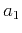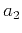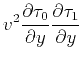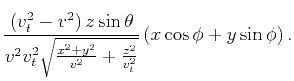Traveltime approximations for transversely isotropic media with an inhomogeneous backgroundNext: Discussion Up: Alkhalifah: TI traveltimes in Previous: The vertical direction

# The symmetry-axis azimuth and the 3-D case

In 3D, the tilt of the symmetry axis is defined by an angle,, measured from vertical, and the azimuth,, of the vertical plane that contains the symmetry axis. Thus,is an angle measured in the horizontal plane from a given axis within that plane. To implement an expansion with respect to, we must considerto be generally small. Since seismic acquisition is often performed in the dip direction of the structure, and we anticipate that the tilt is influenced by the presumed subsurface structure (folding), it would be reasonable to measurefrom the acquisition direction. In this case, I can considerto be small, and thus, approximate the traveltime solution of the eikonal equation with the following expansion:(20)

to be inserted in the acoustic eikonal equation for TI in 3D given by(21)

where,,,, andare stated in Appendix D. This is a complicated eikonal equation that is highly nonlinear, and thus, justifies our effort to simplify it through perturbation theory.

Substituting the trial solution, equation 20, into the eikonal equation for 3D TI media, equation 21, yields a polynomial expansion in the powers of. The zero-order term of this polynomial represents the eikonal equation for TI media for the zero-azimuth case (the 2-D result). The coefficient of theterm yields a first-order PDE for. For simplicity, it is shown here for the case of elliptical anisotropy () as(22)

We can obtain similar PDEs for the other coefficients of the expansion, but obtaining the 2D background TI model will be a challenging task. We can use the 2D version of the equation, developed earlier, to manage this task; however, the influence of ignoring the azimuth on that process could be critical.

A more realistic implementation is achieved by expanding from an elliptically anisotropic background with a vertical symmetry axis background in terms of,, and, simultaneously. However, since a vertical symmetry axis has no particular azimuth (a singularity), I replace the tilt and azimuth byand(and). For simplicity and to be able to include the resulting equations in this paper, I only consider first-order terms of the Taylor's series expansion, and thus, consider the following trial solution:(23)

In Appendix D, we look at higher-order expansions into take advantage of the Shanks transform properties, which considerably helps in thecase. Inserting equation 23 into equation 21 and equating terms of similar powers of the independent parameters (,, and) yields an elliptically anisotropic eikonal equation with a vertical symmetry axis from the zeroth-order term and first order PDEs from the other terms that have the same form as equation 7. The source functions are shown in Appendix D.

Assuming a homogeneous-medium background yields an analytic relation, as shown in Appendix D [equation D-13]:(24)

From this first-order approximation in all three parameters (,, and), we can see that by setting(), the last term in the equation, that contains the tilt component, equals zero. This happens also if(axis is vertical), and the traveltime is dependent only on. The-term (second on the right hand side) in this first-order approximation has no dependence on symmetry angle. Also, note that this equation has a complex variation with offset (i.e. nonhyperbolic), however, reducing to a hyperbolic equation ifand the symmetry angle are equal to zero.Traveltime approximations for transversely isotropic media with an inhomogeneous backgroundNext: Discussion Up: Alkhalifah: TI traveltimes in Previous: The vertical direction

2013-04-02